7 digit number

If 3c54d10 is divisible by 330, what is the sum of c and d?

Result

s1 =  8
s2 =  5
s3 =  11

Solution:Leave us a comment of example and its solution (i.e. if it is still somewhat unclear...):Be the first to comment!Next similar examples: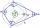What size have angles in quadrilateral (4-gon) if they are in a ratio of 8: 9: 10: 13?
2. Temperature differenceThe highest temperature of Libya was recorded 58 degree Celsius and the lowest was recorded -88 degree Celsius, what is the temperature difference?
3. MO Z8–I–6 2018In the KLMN trapeze, KL has a 40 cm base and an MN of 16 cm. Point P lies on the KL line so that the NP segment divides the trapezoid into two parts with the same area. Find the length of the KP line.
4. N-gon angles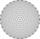What is the sum of interior angles 9-gon? What is the internal angle of a regular convex 9-polygon?
5. KitesBoys run kite on a cable of 68 meters long. What is the kite altitude, if the angle from the horizontal plane is 72°?
6. OctagonWe have a square with side 84 cm. We cut the corners to make his octagon. What will be the side of the octagon?
7. A sculptor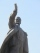A sculptor is duplicating a statue based on an original statue. If the scale factor of the replication is 3.2, will the new statue be larger or smaller than the original statue (enter 1 = larger, 0= smaller)
8. 6 regular polygonIt is given 6 side regular polygon whose side is 5 cm. Calculate its content area. Compare how many more cm2 (square centimeters) has a circle in which is inscribed the 6-gon.
9. Octagon from rectangleFrom tablecloth rectangular shape with dimensions of 4 dm and 8 dm we cuts down the corners in the shape of isosceles triangles. It thus formed an octagon with area 26 dm2. How many dm2 we cuts down?
10. 9-gon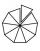Sum of interiol angles of 9-gon is:
11. Hexagon areaThe center of the regular hexagon is 21 cm away from its side. Calculate the hexagon side and its area.
12. Simplify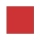Simplify powers multiplication: (3+22)(5-42)
13. The perimeter 2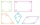The perimeter of the quadrilateral a = 1m b = 14/5m c = 2 3/10m d = 1 4/5m?
14. Hexagon - MOThe picture shows the ABCD square, the EFGD square and the HIJD rectangle. Points J and G lie on the side CD and is true |DJ|
15. Children poolThe bottom of the children's pool is a regular hexagon with a = 60 cm side. The distance of opposing sides is 104 cm, the height of the pool is 45 cm. A) How many liters of water can fit into the pool? B) The pool is made of a double layer of plastic film.
16. 6-gon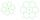Perimeter of regular hexagon is 113. Calculate its circumradius (radius of circumscribed circle).
17. Sphere VSFind the surface and volume of a sphere that has a radius of 2 dm.